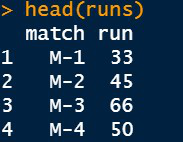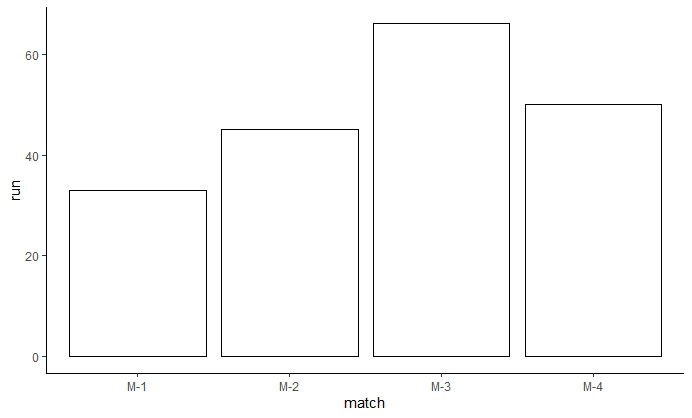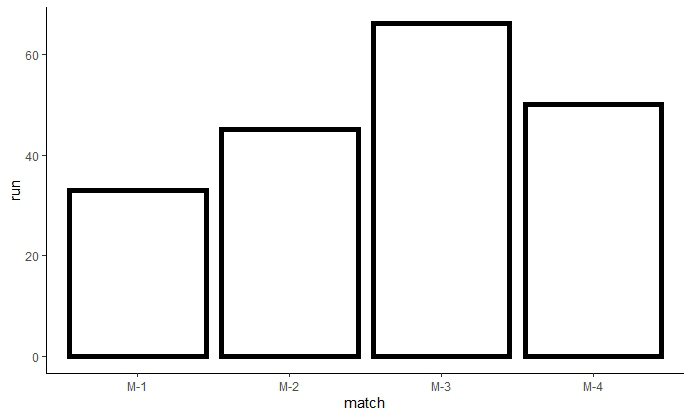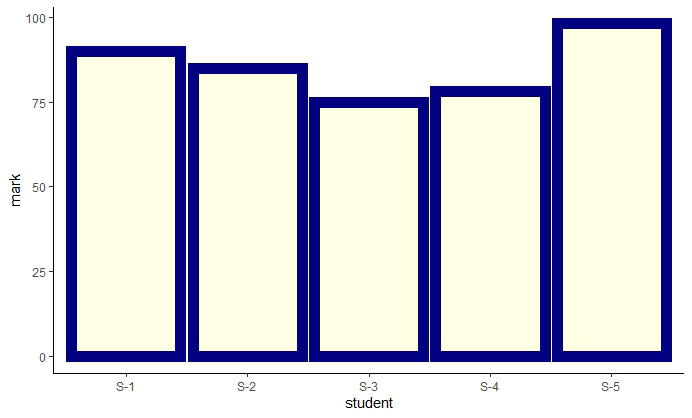# Increase border line thickness of ggplot2 plot in R

• Last Updated : 24 Jun, 2021

In this article, we are going to see how to change the borderline thickness using the ggplot2 bar plot in R Programming Language.

First, you need to install the ggplot2 package if it is not previously installed in R Studio. To install and load write the below command in R Console :

```install.packages("ggplot2")
library(ggplot2)```

For creating a simple bar plot we will use the function geom_bar( ).

Syntax:

geom_bar(stat, fill, color, width)

Parameters :

• stat : Set the stat parameter to identify the mode.
• fill : Represents color inside the bars.
• color : Represents color of outlines of the bars.
• width : Represents width of the bars.

Let’s a Data Frame which has two vectors “match” and “run” and store it in a variable “runs”.

## R

 `# Inserting data``runs <- ``data.frame``(match=``c``(``"M-1"``,``"M-2"``,``"M-3"``,``"M-4"``),``                   ``run=``c``(33, 45, 66, 50))`` ` `head``(runs)`

Output:Bar Plot

## R

 `# Bar Plot ``library``(ggplot2)`` ` ` ` `IPL <-``ggplot``(data=runs, ``aes``(x=match, y=run)) +``  ``geom_bar``(stat=``"identity"``,fill=``"white"``,color=``"black"``)+``  ``theme_classic``()`` ` `IPL`

Output:## Increasing the Border Thickness

Inside the function geom_bar( ), use the keyword size and assign a value to change the thickness of the border. In our case, we have assigned a value of 2 to the size. We can observe that the thickness of the border line has increased.

## R

 `# Changing the border thickness``library``(ggplot2)`` ` ` ` `IPL <-``ggplot``(data=runs, ``aes``(x=match, y=run)) +``  ``geom_bar``(stat=``"identity"``,fill=``"white"``,color=``"black"``,size=2)+``  ``theme_classic``()`` ` `IPL`

Output:Example 2: Consider a Data Frame which consists of information about marks obtained by students in an exam.

## R

 `# Inserting data``marks <- ``data.frame``(student=``c``(``"S-1"``,``"S-2"``,``"S-3"``,``"S-4"``,``"S-5"``),``                   ``mark=``c``(90, 85, 75, 78, 98))`` ` `# Changing the border thickness``library``(ggplot2)`` ` ` ` `RESULT <-``ggplot``(data=marks, ``aes``(x=student, y=mark)) +``  ``geom_bar``(stat=``"identity"``,fill=``"yellow"``,color=``"navy"``,size=4,alpha=0.1)+``  ``theme_classic``()`` ` `RESULT`

Output:My Personal Notes arrow_drop_up